Working with Equations

# 9 Transposing Equations

Click play on the following audio player to listen along as you read this section.Have you ever come across a situation during your math studies where you’re required to solve for a variable which doesn’t seem to be in the right place? Take a look at the following example to see what I mean.

$\Large \text{A} = {\text{B}}^{2} \times 0.7854 \times \text{H}$

In a perfect world, you would like to solve for “A” and at the same time be given the values of both “B” and “H.”But what if you were given “A” and you had to solve for “B”? How would you go about doing this?

The idea here would be to move the variables around and isolate “B.” What this means is that “B” is on one side of the equation by itself, and everything else is on the other side. Take a look at the equation again when this has been done.

$\Large \text{B} = \sqrt{\dfrac{\text{A}}{.7854 \times \text{H}}}$

Changing the formula around is referred to as “transposing” an equation.It’s not as simple as just moving stuff around though. There are rules to get to this point, and those rules and their application are what we are going to deal with in this part of the chapter.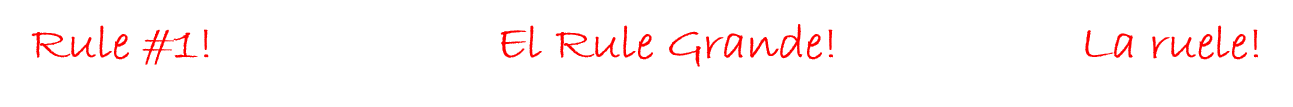The most important thing to remember when transposing equations is that whatever is done to one side of the equation must also be done to the other side of the equation.If you look at it mathematically, this makes sense. We already determined that an equation is two mathematical expressions that are separated by an equal sign.

What this means is the addition, subtraction, division, and multiplication variables and constants on one side of the equation are equal to all the addition, subtraction, division, and multiplication variables on the other side of the equation.

So if you decide to add 5 to one side, you must add 5 to the other side. What this does is keep the equation equal.

Take a look at the following example.

$\Large \begin{array}{c}10+7=9+8 \\ \text{This works out to be:} \\ 17=17\end{array}$

Here we have an equation that is true. Now add 5 to the left hand side of the equation, and you’ll see that, in order to keep the equation true, you’ll have to add 5 to the right hand side of the equation.

$\Large \begin{array}{c} 10 + 7 + \mathbf{5} = 9 + 8 + \textbf{?} \\ 22 = 9 + 8 + ? \\ 22 = 9 + 8 + \mathbf{5} \\ 22 = 22 \end{array}$Keep in mind that this is an example where we added to one side. If we had subtracted, divided, or multiplied, things would be different. We would have to do the same thing to the other side.

# Transposing Equations Using Addition and Subtraction

$\Large \begin{array}{c}7+2=8+1 \\ \text{This works out to be:}\\ 9=9\end{array}$

Then add 4 to one side of the equation and solve.

$\Large 7+2+4=8+1+ \text{ ?}$

As the left hand side of the equation has had 4 added to it, the right hand side of the equation also has to have 4 added to it. We get:

$\Large \begin{array}{c}7+2+ \mathbf{4} = 8 + 1 + \textbf{?} \\ 13 = 9 + \text{ ?} \\ 13 = 9 + \mathbf{4} \\ 13 =13 \end{array}$As stated before, the rule is that whatever you do to one side you must do to the other. In this case, if we add 4 to one side then we have to add 4 to the other side to keep it equal.

Subtraction would work the same way. If we were to subtract 4 from one side we would have had to subtract 4 from the other side in order to keep it equal.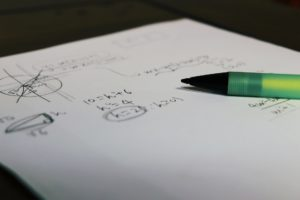Let’s try out this concept with an equation that has an unknown variable in it. Take a look at the following equation and solve for “J.”

$\Large 7+ \text{J}=5+8$

In order to solve for “J” we must isolate “J” on one side of the equation, and it actually doesn’t matter which side we isolate “J” on. If we follow our rule, in order to isolate “J” we would have to get rid of the 7 on the left side. The question becomes, how is that done? What we essentially have to do is move the 7 from the left side to the right side.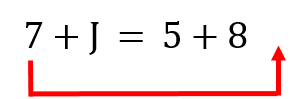Once again, we always have to keep in mind that whatever we do to one side, we must do to the other.

Start with this. Would you agree that 7 − 7 = 0?

What if we subtracted 7 from the left hand side of the equation? What we would be left with is just “J” on the left side, which solves the problem of isolating “J.”

$\Large \begin{array}{c} \mathbf{(7-7)} +\text{J} = 5+8 \\ 0 + \text{J} = 5+8 \\ \text{J}= 5+8 \end{array}$

Mathematically this is not correct yet as we only dealt with the left side of the equation. What we need to do now is to do the same thing to the right side and then solve the equation. So we end up subtracting 7 from the right side of the equation to make everything equal once again.

$\Large \begin{array}{c}(7-7)+\text{J} = 5+8-7 \\ 0 + \text{J} = 13-7 \\ \text{J} = 13 - 7 \\ \text{J}=6 \end{array}$You can always check that your answer is correct by taking the answer and putting it back in the equation to replace the variable.

$\Large \begin{array}{rl}\text{Replace J with 6} & \\ \downarrow & \\ 7 + \text{J} & = 5+8 \\ 7+6 & = 5+8 \\ 13 & = 13 \end{array}$ExampleSolve for G.

$\Large 27+ \text{G} = 43+49$

Step 1: Isolate the variable you are trying to find. In this case “G.” In order to do this, we must remove the 27 from the left side of the equation. This is done by subtracting 27 from the left side and then also from the right side.

$\Large \begin{array}{c}27+\text{G} = 43+49 \\ \mathbf{(27-27)}+\text{G} = 43+49 - \mathbf{27}\end{array}$

Step 2: Work through the equation.

$\Large \begin{array}{c}(27-27) +\text{G} = 43+49-27 \\ 0 + \text{G} = 92-27 \\ \text{G}=65\end{array}$

$\Large \begin{array}{rl}\text{Replace G with 65} &\\ \downarrow & \\ 27 + \text{G} & = 43 + 49 \\ 27 + 65 & = 43 + 49 \\ 92 & =92\end{array}$ExampleSolve for H.

$\Large \text{H}-16=13+19$

Step 1: Isolate the variable you are trying to find. In this case “H.” In order to do this we must remove the 16 from the left side of
the equation. This is done by adding 16 to the left hand side of the equation and then also adding 16 to the right hand side of
the equation.

$\Large \begin{array}{c}\text{H}-16 = 13+19 \\ \text{H} + \mathbf{(-16+16)}=13 + 19 \mathbf{ +16}\end{array}$

Step 2: Work through the equation.

$\Large \begin{array}{c}\text{H}+(-16+16)=13+19+16 \\ \text{H}+0=32+16 \\ \text{H}=48 \end{array}$

$\Large \begin{array}{l}\text{Replace H with 48} \\ \downarrow \\ \text{H}-16 = 13+19 \end{array}$

$\Large \begin{array}{c} 48-16=13+19 \\32=32 \end{array}$# Practice Questions

Now it’s time to do a couple of practice questions for yourself. Make sure to check the video answers to see how you did.

Question 1Solve for S.

$\Large 142+\text{S}=198+257$

Question 2Solve for Y.

$\Large \text{Y} - 22 = 51+53$

# Transposing Equations Using Multiplication and Division

Transposing equations using multiplication and division uses the same basic principle as addition and subtraction in that whatever you do to one side you must do to the other.

Before we dive into this part of the chapter, we should refresh our memories a little and talk about reciprocals as it relates to math. Take a look at the following two numbers with one being a whole number and the other a fraction.

$\Large 4 \text{ and } \dfrac{1}{4}$

Don’t forget that when we write the number 4, we could also write it as:

$\Large\dfrac{4}{1}$

Do you remember what will happen if we multiplied those two together?

$\Large 4 \times \dfrac{1}{4} = \dfrac{4}{4} = 1$

Reciprocals are numbers that when multiplied together equal 1. This is an important concept when transposing using multiplication and division. If we divide a number by its reciprocal and end up with one, we have essentially removed that number from the equation.

Here is an example. Solve for K.

$\Large 8 \times \text{K} = 14 \times 17$

Similar to transposing equations with addition and subtraction, the first thing to do is to isolate the variable we are trying to find. In this case, “K.” This means that the 8 needs to be removed from the left hand side of the equation. Multiplying 8 by its reciprocal will give us a value of 1. Perfect!

$\Large 8 \times \dfrac{1}{8} = \dfrac{8}{8} = 1$

Now adding this information to the equation, we would end up with this:

$\Large \begin{array}{c} 8 \times \dfrac{1}{8} = \dfrac{8}{8} = 1 \\ \mathbf{\dfrac{1}{8}} \times 8 \times \text{K}= 14 \times 17 \\ \dfrac{8}{8} \times \text{K} = 14 \times 17\\ 1\times \text{K}=14\times 17 \\ \text{K}=14\times 17 \end{array}$

This would leave us with 1 × K on the left hand side of the equation which would end up being just “K” when multiplied together. This is exactly what we are looking for. We are not quite finished yet though. Now back to the golden rule.

Whatever you do to one side of the equation you must do to the other. Therefore, as we multiplied the left hand side of the equation by we need to multiply the right hand side of the equation by .

$\Large \dfrac{1}{8} \times 8 \times \text{K} = 14 \times 17 \times \dfrac{1}{8}$

So now if we followed this through we would end up with:

$\Large \begin{array}\\ \mathbf{\dfrac{1}{8}} \times 8 \times \text{K} = 14 \times 17 \times \mathbf{ \dfrac{1}{8}} \\ \dfrac{8}{8} \times \text{K} = \dfrac{238}{8} \\ \text{K} = 29.75 \end{array}$

We should do a check of the answer like we did previously to see if we are correct.

$\Large \begin{array}{rl}\text{Replace K with 29.75} & \\ \downarrow & \\ 8 \times \text{K} & = 14 \times 17 \\ 8 \times 29.75 & = 14 \times 17 \\ 238 & = 238\end{array}$Example

We’ll try another example, but this time we’ll deal with fractions that will have to be moved around. We’ll also start to do this in steps as we have done with previous questions.

Solve for L.

$\Large \dfrac{4}{9} \times \text{L} = 12 \times 12$

Step 1:  Isolate L. In this case, we have to move the 4/9 from one side to the other. In order to do this we have to multiply both sides by the reciprocal of 4/9. This will essentially remove 4/9 from the left hand side of the equation. The reciprocal is of 4/9 is 9/4.

$\Large \dfrac{4}{9} \times \dfrac{9}{4} = \dfrac{36}{36} = 1$

Therefore we get:

$\Large \dfrac{4}{9} \times \dfrac{9}{4} \times \text{L} = 12 \times 12 \times \dfrac{9}{4}$

Step 2: Solve the equation

$\Large \begin{array}{c} \dfrac{4}{9} \times \dfrac{9}{4} \times \text{L} = 12 \times 12 \times \dfrac{9}{4} \\ 1 \times \text{L} = 144 \times \dfrac{9}{4} \\ \text{L} = \dfrac{1296}{4} \\ \text{L}=324 \end{array}$

$\Large \begin{array}{rl}\text{Replace L with 324} & \\ \downarrow & \\ \dfrac{4}{9} \times \text{L} & = 12 \times 12 \\ \dfrac{4}{9} \times 324 & = 12 \times 12 \\ \dfrac{1296}{9} & = 144 \\ 144 & = 144 \end{array}$Example

This one is a bit more challenging. You’ll note in the question that there are no numbers, only letters. Take a look at the question, and see if you can come up with any ideas on how you would solve this equation.

Solve for “D” in the following equation.

$\Large \dfrac{\text{A}}{\text{B}}= \dfrac{\text{C}}{\text{D}}$

Step 1:  Determine which variable you must isolate. In this case it’s given for us and it’s “D.”

$\Large \dfrac{\text{A}}{\text{B}}= \dfrac{\text{C}}{\textbf{D}}$

The challenge here is that “D” is in the denominator (bottom) of the fraction. If we were to isolate it but it was still in the denominator of a fraction we would not have something that we could work with.

When “D” is isolated it must not only be by itself on one side of the equation but also appear as a whole number and not as the denominator of a fraction.

Step 2: To make this process easier break the equation down to look like the following.

$\Large \dfrac{\text{A}}{1} \times \dfrac{1}{\text{B}}= \dfrac{\text{C}}{1} \times \dfrac{1}{\text{D}}$

Remember that:

$\Large \dfrac{\text{A}}{1} \times \dfrac{1}{\text{B}}= \dfrac{\text{A}}{\text{B}}$

We haven’t really changed anything mathematically we have just made the equation into something that is easier to work with.

Step 3:  Isolate “D.” In order to do this we must remove “C” from the right hand side of the equation. Do this by multiplying both the right and left hand side by 1/C.

$\Large \mathbf{\dfrac{1}{\text{C}}} \times \dfrac{\text{A}}{1} \times \dfrac{1}{\text{B}}= \dfrac{\text{C}}{1} \times \dfrac{1}{\text{D}} \times \mathbf{\dfrac{1}{\text{C}}}$

Now this might start looking a little bit complicated but if you follow it through you’ll start to see the answer form. Look at the right hand side of the equation. It now has both and C/1 and a 1/C. Multiplying those reciprocals together you get 1 and effectively take out the “C” on the right hand side.

$\Large \dfrac{\text{C}}{1} \times \dfrac{1}{\text{C}}= \dfrac{\text{C}}{\text{C}}=1$

So we end up with:

$\Large \dfrac{1}{\text{C}} \times \dfrac{\text{A}}{1} \times \dfrac{1}{\text{B}}= 1 \times \dfrac{1}{\text{D}}$

If we wanted to simplify this we could just do the following:

$\Large \dfrac{\text{A}}{\text{C} \times \text{B}} = \dfrac{1}{\text{D}}$

Step 4: Get D into the numerator of the equation. What we need to do will take a little bit of math and some patience. We need to follow the same rules that we have been following.

The idea here is to multiply each side by D/1. This will make the right hand side of the equation equal to 1 but now the left hand side of the equation will have D in the numerator.

$\Large \begin{array}{c}\dfrac{\text{D}}{1} \times \dfrac{\text{A}}{\text{C} \times \text{B}} = \dfrac{1}{\text{D}} \times \dfrac{\text{D}}{1} \\ \dfrac{\text{D}}{1} \times \dfrac{\text{A}}{\text{C}\times \text{B}} = 1 \end{array}$

What you’ll notice is that it actually creates more work but we have managed to get “D” into the numerator of the equation. The only problem is that we also have a bunch of other stuff on the same side as “D” and now our job becomes getting rid of all that stuff.

Now all we have to do is follow the rules and get the A, B and C over to the right hand side and isolate D. I’ll do this simply and in one quick calculation.

$\Large \dfrac{\text{D}}{1} \times \dfrac{\text{A}}{\text{C} \times \text{B}} \times \dfrac{\text{C} \times \text{B}}{\text{A}} = \dfrac{\text{C} \times \text{B}}{\text{A}}$

What we end up with is:

$\Large \text{D}= \dfrac{\text{C} \times \text{B}}{\text{A}}$

There is a lot of math involved there but if you follow the rules and go one step at a time you’ll eventually get there. My suggestion here is that you go over what we just went through a couple times before moving on. Understanding the math involved here is quite important when it comes to transposing formulas and equations.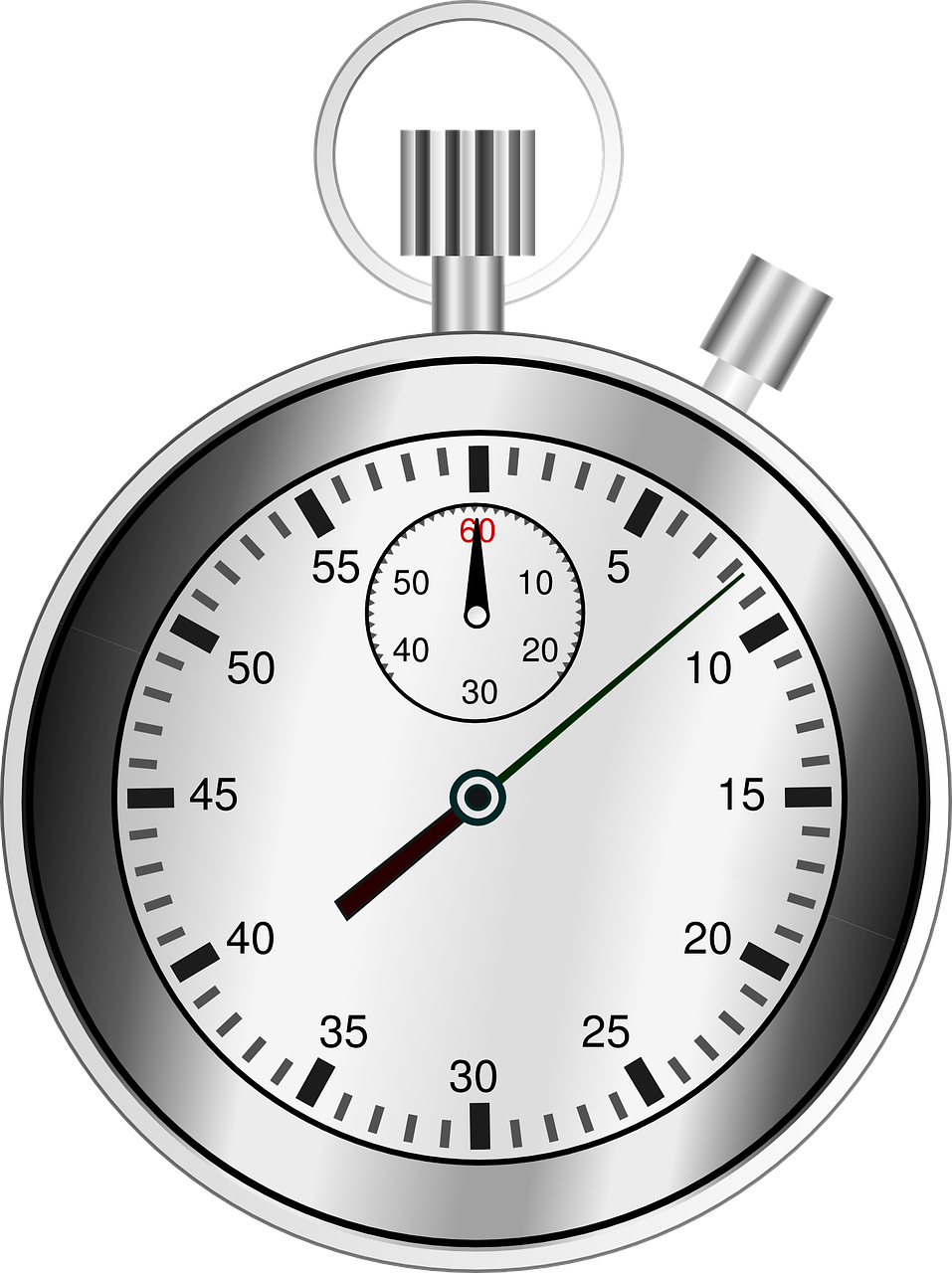At this point you might be wondering if there was a shortcut and as luck would have it there is for this procedure which I’ll go through now.. We’ll start with an example.

Take the following equation:

$\Large \dfrac{3}{4}=\dfrac{3}{4}$

This equation is true. Now take each of the fractions and flip them around.

$\Large \dfrac{4}{3} = \dfrac{4}{3}$

It seems that if you flip both fractions around then the equation is still true. Mathematically you are doing the same thing to one side as you are doing to the other. There is a lot of math involved here but the main point is that if you were to do all the math you would end up with the same answer.

How we can use this to help us simplify our question is to just do the following. Remember we started with:

$\Large \dfrac{\text{A}}{\text{B}}= \dfrac{\text{C}}{\text{D}}$

We needed to isolate D but the main problem (and the thing that causes us to do a lot of work) was that D was in the denominator and we needed it in the numerator.

Through our math wizardry we can get D into the numerator by just flipping around the left hand fraction. Whatever we do to that side we then must do to the right side. What we end up with is:

$\Large \dfrac{\text{B}}{\text{A}}= \dfrac{\text{D}}{\text{C}}$

All we need to do now is get C out of the left hand side by multiplying it by C/1 and then doing the same thing to the left hand side.

$\Large \dfrac{\text{C}}{1} \times \dfrac{\text{B}}{\text{A}}= \dfrac{\text{D}}{\text{C}} \times \dfrac{\text{D}}{1}$

We end up with…

$\Large \dfrac{\text{C} \times \text{B}}{\text{A}} = \text{D}$

Now to take all that in in one shot might be a little much so you may want to go back and reread that whole explanation. But always keep in mind the math that goes along with that.

ExampleIn this example, we are going to use the formula for the area of a circle. Calculating the area of a circle is actually something we are going to do further into this volume, but for now we are just going to deal with the formula itself.

$\Large \begin{array}{c} \text{Area of a circle} \\ \text{A} = {\text{D}}^{2} \times 0.7854 \end{array}$

$\Large \begin{array}{cl} \text{Where:} & \text{A = area of the circle} \\ & \text{D = diameter of the circle} \end{array}$

Solving for the area of the circle would be pretty straight forward but as we are dealing with transposing equations in this section what we’ll do is solve for the diameter (D).

Step 1: Identify the variable you are trying to solve for.

$\Large \text{A} = \mathbf{{\text{D}}^{2}} \times 0.7854$

From this you can see we have a couple problems we have to deal with. The first one is that we have to isolate D. The second one is that D has the exponent 2 attached to it so we have to somehow eliminate that.

Step 2: Isolate D.

For this we have to move the 0.7854 from the right hand side of the equation to the left hand side. We simply follow the rules we have used up to this point.

$\Large \begin{array}{c} \mathbf{\dfrac{1}{0.7854}} \times \text{A} = {\text{D}}^{2} \times 0.7854 \times \mathbf{\dfrac{1}{0.7854}} \\ \dfrac{\text{A}}{0.7854} = {\text{D}}^{2} \times \dfrac{0.7854}{0.7854} \\ \dfrac{\text{A}}{0.7854} = {\text{D}}^{2} \times 1 \\ \dfrac{\text{A}}{0.7854} = {\text{D}}^{2} \end{array}$

That takes care of the first problem. Now we have to tackle the D2 issue.

Step 3: Remove the exponent from D.

Once again we go back to our original rule. Whatever you do to one side you must to the same thing to the other side.

We have to refer to the video that you watched earlier in this section regarding exponents and square roots. We’ll do a quick refresher before we solve for D.

Remember that:

$\Large \begin{array}{c} {\text{D}}^{2} = \text{D} \times \text{D} \\ \text{and... } \\ \sqrt{\text{D} \times \text{D}} = \text{D} \end{array}$

So what we see is that if you square root a number that is squared (has an exponent of 2) you end up with the number itself. I’ll quickly show you with numbers.

$\Large \begin{array}{c} {4}^{2} = 4 \times 4 \\ {4}^{2} = 16 \\ \sqrt{16} = \sqrt{4 \times 4} \\ \sqrt{16} = 4 \end{array}$

Having gone through all that we can now solve the problem.

$\Large \begin{array}{c} \sqrt{\dfrac{\text{A}}{0.7854}}= \sqrt{{\text{D}}^{2}} \\ \sqrt{\dfrac{\text{A}}{0.7854}} = \text{D} \end{array}$

# Practice Questions

Now it’s time to do a couple of practice questions for yourself. Make sure to check the videos answer to see how you did.

Question 1Solve for B.

$\Large \dfrac{\text{A} \times \text{B}}{\text{C}}=\dfrac{\text{D} \times \text{E}}{\text{F}}$

Question 2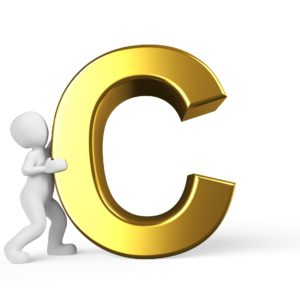Solve for C.

$\Large \dfrac{\text{A} \times \text{B}}{\text{C}}=\dfrac{\text{D} \times \text{E}}{\text{F}}$